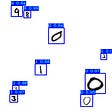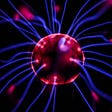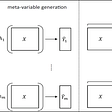DiDiffEqFlux — Differential Equations and Neural Networks

What I cannot create, I do not understand”-Richard Feynman

I’ve been thinking about ways to approximate the dynamics behind data. Classification methods including neural networks and logistic regression work but I wanted to learn a different, possibly better, way. I’ve been following Chris Rackackaus, PhD, for some time now. He teaches at MIT and also does a lot of work with differential equations using Julia, which is the primary language that I use to program and model things. He has really contributed a lot to DiffEqFlux. To summarize DiffEqFlux it is a package that allows the user to fit universal differential equations using neural networks. The neural networks can be a part of the differential equations and solved, or, if there is only data that you have and want to find a way to model it, parameters can be found which can help to approximate your function. I have chosen to demonstrate the latter using DiffEqFlux.

A lot of the demonstrations and tutorials used for DiffEqFlux were showing how to solve a differential equation where the equation was known such as the Lotka Volterra equations (the bunnies and wolves). Others used data that was simulated and tried to approximate the parameters for both the diffusion and drift (stochastic differential equations). I attended an online Meetup with the Stuttgart Julia Programming Language Group (shoutout to Maren!). There the main speaker demonstrated how she was modeling her data as a molecular biologist and finding a way to approximate her data with parameters found using DiffEqFlux. I wanted to look into how to do this so that I could understand it better and use it.

The function I wanted to model was the exponential equation

To have this be a true stochastic equation I chose to add some noise to my data. My data was from x=-3 to x=3 with random noise added in:

`sde_data=[exp(x) for  x in -3:3]noise_amount=0 .+2*rand(length(sde_data))sde_data=sde_data+noise_amount`

As mentioned earlier there are parameters for both the drift and the diffusion.

To have the program move faster the layers will have, at most, 20 nodes to them. One is for the fixed diffusion term and the other is for the random part as a Wiener process.

`fixed = FastChain((x,p)->x.^3,        FastDense(3,20,sigmoid),        FastDense(20,20,sigmoid),        FastDense(20, 3))wiener = FastChain(FastDense(3, 20, sigmoid),         FastDense(20, 3))`

The problems are defined as an ODE, PDE or SDE. This demonstration is an SDE and is repesented as one with diagonal noise:

`neuralsde = NeuralDSDE(fixed, wiener, tspan,SOSRI(),            sensealg=TrackerAdjoint(),saveat = tsteps,             reltol = 1e-1, abstol = 1e-1,            allow_f_increases=true)`

Now for some fun!

We run the training for 500 epochs first. One great part of using DiffEqFlux is that much of the existing framework from Flux.jl is used in tandem. There are callback funcitons and the same optimizers can be used (mostly ADAM I mean who are we kidding!). The resulting graph of the fit is:

This could be better. The loss function uses mean squared error to minimize the distance between the prediction and the data. It could have either been caught in a local minima which doesn’t seem to be the case as the prediction more or less follows the general flow of our data, or more training epochs could have helped. The loss is shown as

There was was a minimum loss achieved at 200 epochs and again at 500 epochs. Doesn’t seem that more training would help. Now we can adjust the time periods or the amount of data points that are trained by our networks. We start with a certain number of data points and then add one or two, train the networks on those, add one or two more and keep going until the model is trained on all of the data points. This strategy is useful for breaking out of a local minima. The resulting graph was trained on 2 points to start and adds one in each time as our set was small but if it was very large more would be added at a time. Each addition was trained for 300 iterations with each separate point and the resulting fit was shown as:

Much better! Especially in the beginning of our data. More training might have helped and we see that we can actually get pretty close to approximating our function. The more noise in the function over the time series (think a stock price) the worse of a fit that the model can have. Some data is really random and will have to be planned for. If your data does have a certain repetition for it then it will be easier to model. The more randomness that there is in it then the harder it will be. This demonstration was for making sense of data and not so much to approximate the parameters of known functions over a certain time period but how close we can get to reproducing the results/datapoints that we have. There is some preprocessing involved such as normalization (not here to keep the data a little messier), starting the data at (0,0) etc.

Link to the repository is here:

--

--

--

Studying applied math and programming my way through life.

Love podcasts or audiobooks? Learn on the go with our new app.

## Train & Test Models in Google Cloud + Ludwig with no code## Tame Any Sequence with Transformers## Blending the Blender for NLG tasks ?## Mathematics In Machine Learning## TensorFlow 2 YOLOv3 Mnist detection training tutorial## Machine Learning Compass## “Hotdog or not hotdog” app with IBM Cloud## Step-By-Step Building A Neural Network From ScratchStudying applied math and programming my way through life.

## Variational Autoencoder : Intuition and Implementation## Introducing a Multi-target regression## Attributable Risk and Interval Estimation for Distributed Lag Models## Introduction to Machine Learning Ensemble Methods(Part 1/2)